International
Tables for
Crystallography
Volume F
Crystallography of biological macromolecules
Edited by M. G. Rossmann and E. Arnold

International Tables for Crystallography (2006). Vol. F, ch. 13.2, p. 273   | 1 | 2 |

## Section 13.2.5. Other rotation functions

J. Navazaa*

aLaboratoire de Génétique des Virus, CNRS-GIF, 1. Avenue de la Terrasse, 91198 Gif-sur-Yvette, France
Correspondence e-mail: jnavaza@pasteur.fr

### 13.2.5. Other rotation functions

| top | pdf |

The rotation function was hitherto described in terms of self- and cross-Patterson vectors. This is perhaps inevitable in the self-rotation case, but the problem of determining the absolute orientation of the subunits when a model structure is available may be formulated in a different way. We may try to compare directly the observed and calculated intensities or structure factors by using any criterion analogous to those employed in refinement procedures, e.g., the crystallographic R factor or correlation coefficients.

When the space-group symmetry is explicitly exhibited, the structure factor corresponding to a crystal with M independent molecules in the unit cell takes the form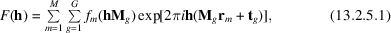where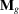anddenote, respectively, the transformation matrix and the translation associated with the gth symmetry operation of the crystal space group. The corresponding intensity is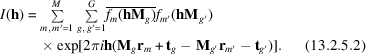For criteria based on amplitudes, the calculated structure factor will contain only the contribution of the rotated model,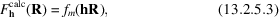i.e., the Fourier transform of a single molecule in the crystal cell, assuming P1 symmetry. For criteria based on intensities, some symmetry information may be introduced,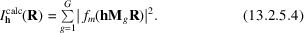A criterion often considered is the correlation coefficient on intensities,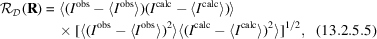where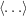means `average over reflections'. It may be calculated within reasonable computing time provided that

 (1) the structure factors are computed by interpolation from the Fourier transform of the isolated molecule's electron density; and (2) an efficient sampling set of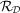is defined.is referred to as the direct-rotation function (DeLano & Brünger, 1995). A major advantage of this formulation is that the information stemming from already-positioned subunits may be taken into account, just by adding their contribution to the calculated intensities.

### References

DeLano, W. L. & Brünger, A. T. (1995). The direct rotation function: rotational Patterson correlation search applied to molecular replacement. Acta Cryst. D51, 740–748.Google Scholar